# 五行代码用图提升模型表现，TensorFlow开源NSL神经结构学习框架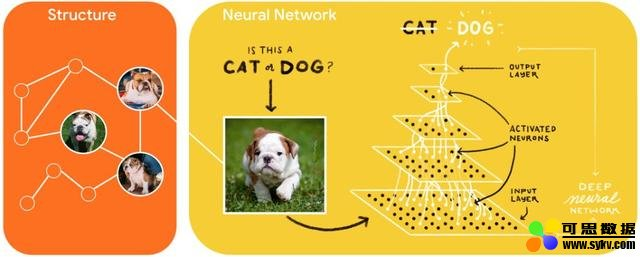• 项目地址：https://github.com/tensorflow/neural-structured-learning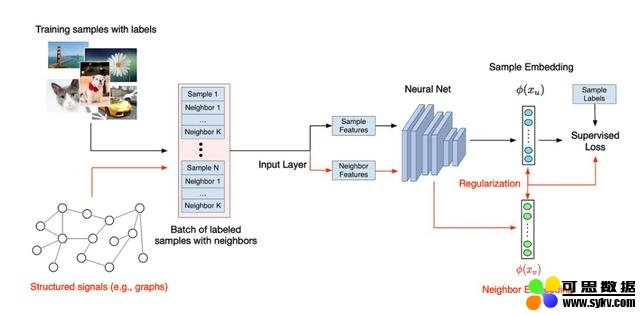NSL 让 TensorFlow 用户能够轻松地结合各种结构化信号来训练神经网络，且适用于不同的学习场景：监督、半监督和无监督（表示）设置。 可思数据-AI,sykv.com智能驾驶,人脸识别,区块链,大数据

NSL 如何工作 可思数据sykv.com,sykv.cn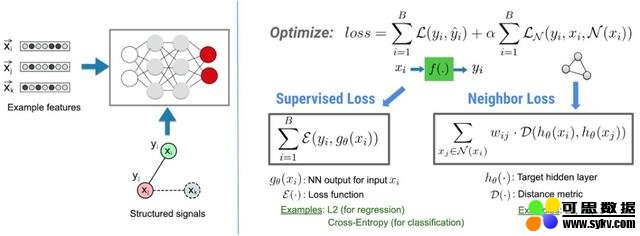NSL 的基本架构。

` 可思数据-数据挖掘,智慧医疗,机器视觉,机器人sykv.com `
1. python pack_nbrs.py --max_nbrs=5 \
2. labeled_data.tfr \
3. unlabeled_data.tfr \
4. graph.tsv \
5. merged_examples.tfr

` 可思数据-AI,sykv.com人工智能,深度学习,机器学习,神经网络 `
1. import neural_structured_learning as nsl
2. # Create a custom model — sequential, functional, or subclass.
3. base_model = tf.keras.Sequential(…)
4. # Wrap the custom model with graph regularization.
5. graph_config = nsl.configs.GraphRegConfig(
6.  neighbor_config=nsl.configs.GraphNeighborConfig(max_neighbors=1))
7. graph_model = nsl.keras.GraphRegularization(base_model, graph_config)
8. # Compile, train, and evaluate.
10.  loss=tf.keras.losses.SparseCategoricalCrossentropy(), metrics=[‘accuracy’])
11. graph_model.fit(train_dataset, epochs=5)
12. graph_model.evaluate(test_dataset)

` 可思数据sykv.com,sykv.cn `
1. import neural_structured_learning as nsl # Create a custom model — sequential, functional, or subclass.
2. base_model = tf.keras.Sequential(…)# Wrap the custom model with graph regularization.
3. graph_config = nsl.configs.GraphRegConfig(
4.  neighbor_config = nsl.configs.GraphNeighborConfig(max_neighbors=1))
5. graph_model = nsl.keras.GraphRegularization(base_model, graph_config) # Compile, train, and evaluate.
7.  loss=tf.keras.losses.SparseCategoricalCrossentropy(), metrics=[‘accuracy’])
8. graph_model.fit(train_dataset, epochs=5)
9. graph_model.evaluate(test_dataset)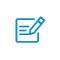扫码入群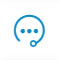咨询反馈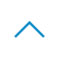返回顶部